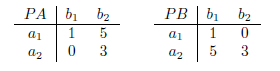## Nash Equilibrium

• 문제 ID
• 시간 제한
• 메모리 제한
• 제출 횟수
• 정답 횟수 (비율)
• 출처
• 분류

#### 문제

A two-player normal form game between two individuals A and B is completely specified by

• {a1, . . . , am}, a set of actions for player A,
• {b1, . . . , bn}, a set of actions for player B,
• PA, an m× n payoff matrix for player A, and
• PB, an m× n payoff matrix for player B.

In such a game, both players simultaneously select actions to be played (say ai and bj for players A and B, respectively). Then payoffs for each player are determined according to the payoff matrices (PA[i, j] and PB[i, j] for players A and B, respectively). The goal of each player is to maximize his or her payoff.
For player A, the set of best responses to a particular action bj by player B consists of any action ai which maximizes A’s payoff, that is, whose payoff is maxi0PA[i0, j]. Similarly, for player B, the set of best responses to a particular action ai by player A is any action bj whose payoff is maxj0PB[i, j0]. A pair of strategies (ai, bj) is said to be a pure strategy Nash equilibrium if ai is a best response to bj and bj is a best response to ai.
In this problem, you are given the payoff matrices for two players A and B, and your task is to find and list all pure strategy Nash equilibria.

For an example, consider the following two-player game in which A and B each have two possible actions, and the payoff matrices are:Here, if player A chooses a1, then choosing b1 allows player B to maximize his payoff (PB[1, 1] = 1 > 0 = PB[1, 2]). Similarly, if player B choose b1, then choosing a1 allows player A to maximize his payoff (PA[1, 1] = 1 > 0 = PA[2, 1]). Thus, a1 is the best response for b1 and vice versa, so (a1, b1) is a pure strategy Nash equilibrium of this game. However, note that (a2, b2) is not a Nash equilibrium; if player A chooses action a2, b1 is the best response since PB[2, 1] = 5 > 3 = PB[2, 2].

#### 입력

The input test file will contain multiple test cases. Each test case begins with a single line containing two integers, m and n, where 1  m, n  20. The next m lines specify the m rows of payoff matrix PA. The m lines after that specify the m rows of payoff matrix PB. All payoff matrix values will be integers between -100 and 100, inclusive. The end-of-file is marked by a test case with m = n = 0 and should not be processed.

#### 출력

For each input case, suppose that N is the number of Nash equilibria for the described normal form game. Then, the output of the program consists of (1) a line containing the single integer N, and (2) N lines containing two integers i and j, where (ai, bj) is the corresponding Nash equilibrium. Note that the program must list all Nash equilibria in lexicographical order, i.e., (ai1 , bj1 ) is listed before (ai2 , bj2 ) if i1 < i2 or if i1 = i2 and j1 < j2.

#### 예제 입력

3 4
1 5 -3 2
4 2 -1 -3
3 -2 5 9
0 -4 -1 -5
-9 2 8 3
-3 4 -2 3
2 2
1 10
0 5
1 0
10 5
2 2
1 1
1 1
1 1
1 1
0 0

#### 예제 출력

0
1
1 1
4
1 1
1 2
2 1
2 2